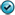## Exercises - Intermediate Value Theorem

1. Decide whether or not the Intermediate Value Theorem can be applied to the given function, value, and interval. Justify your decision carefully. When this theorem applies, state carefully what it concludes. Finally, find all values $c$ in the given interval, such that $f(c)=k$.

1. $f\,(x) = x^2-2x+1; \quad k=4, \ [-3,2]$

2. $f\,(x) = 4x^4-9; \quad k=0, \ [1,2]$3. $f\,(x) = -x^2 + 3x; \quad k=0, \ [-1,2]$

4. $f\,(x) = x^2 + x + 1; \quad k=7, \ [-2,3]$

5. $\displaystyle{f\,(x)=\frac{10}{x^2+1}; \quad k=8, \ [0,1]}$

6. $f\,(x) = \sqrt{x+3}; \quad k=2, \ [-3,6]$

7. $\displaystyle{f\,(x) = \frac{1}{2x-1}; \quad k=0.5, \ [1,2]}$

8. $\displaystyle{f\,(x) = \frac{x^2+8}{x}; \quad k=6, \ [1,3]}$